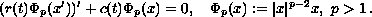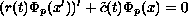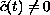Fourth Mississippi State Conference on Differential Equations and Computational Simulations,
Electron. J. Diff. Eqns., Conf. 03, 1999, pp. 29-37.

### A remark on the half-linear extension of the Hartman-Wintner theorem Ondrej Dosly

Abstract:
We establish a Hartman-Wintner type theorem for the half-linear second order differential equationThis equation is viewed as a perturbation of the non-oscillatory equationwitheventually.

Published July 10, 2000.
Mathematics Subject Classifications: 34C10.
Key words: Half-linear equation, scalar p-Laplacian, Hartman-Wintner criterion.

Show me the PDF file (116K), TEX file, and other files for this article.Ondrej Dosly Mathematical Institute, Czech Academy of Sciences, Zizkova 22, CZ-616 62 Brno email: dosly@math.muni.cz